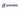# How to reorder columns in Pandas DataFrameShare

There are different methods we can use to reorder DataFrame columns in Pandas using Python. The easiest way to change the order of columns in Pandas are:

Let’s get started creating a Pandas DataFrame that we will manipulate in the next chapters. To do so, we can simply use the following Python code:

``df = pd.DataFrame(np.random.rand(10, 4), columns=['A', 'C', 'B', 'D'])``

The output should be something similar to this:

## Change the order of DataFrame columns using double square brakets

In Pandas, we can use the square brackets to select a particular subset of columns. For example, if we want to see only the columns “A” and “B” we can use the following code:

``df[['A', 'B']]``

When we use the square brackets the returned value is a new DataFrame with the selected columns in that specific order. So, if we want to change the order of the columns in a Pandas DataFrame using square brackets we can use the following piece of Python code:

• `df = pd.DataFrame(np.random.rand(10, 4), columns=['A', 'C', 'B', 'D'])`
• `df = df[['A', 'B', 'C', 'D']]`

In the example above we created a DataFrame with columns “A”, “C”, “B”, and “D” containing random numbers and changed the order of the columns in “A”, “B”, “C” and “D”.

## Reorder Pandas columns using pandas.DataFrame.reindex

Another method to reorder the DataFrame columns in Pandas is by using the function `pandas.DataFrame.reindex`. We can call the `reindex()` function passing into the `columns` parameter a list containing the new order of columns. In this case, if we want to change the order of the columns using `pandas.DataFrame.reindex` we can use the following Python code:

• `df = pd.DataFrame(np.random.rand(10, 4), columns=['A', 'C', 'B', 'D'])`
• `df = df.reindex(columns=['A', 'B', 'C', 'D'])`

Easy! The result is the same as before: we changed the column’s order in “A”, “B”, “C” and “D”.